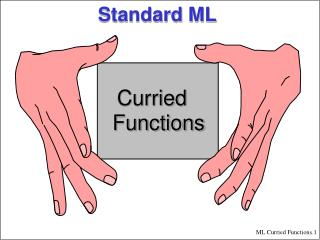DownloadDownload PresentationStandard ML

# Standard ML

Télécharger la présentation## Standard ML

- - - - - - - - - - - - - - - - - - - - - - - - - - - E N D - - - - - - - - - - - - - - - - - - - - - - - - - - -
##### Presentation Transcript

1. Standard ML Curried Functions

2. Curried Functions • Side Note: Infix function declaration • Curried Function declaration • Declaring via "fun" • Function Calling Order • Examples • Mixing infix & curried

3. Side Note - Operators • A function of two arguments can be treated using infix notation fun d(x,y) = Math.sqrt(x*x+y*y); val d = fn : real * real -> real - d; val it = fn : real * real -> real - d(1.0,3.0); val it = 3.16227766017 : real • Convert to infix - infix d; infix d - 1.0 d 3.0; val it = 3.16227766017 : real - 1.0 d 3.0 d 2.0 d 5.0; val it = 6.2449979984 : real

4. Operators - a bit more • Access - d; stdIn:40.1 Error: expression or pattern begins with infix identifier "d" - op d; val it = fn : real * real -> real - op d(1.0,3.0); val it = 3.16227766017 : real Infix declaration can come before function definition - infix d; infix d - fun x d y = Math.sqrt(x*x + y*y); val d = fn : real * real -> real

5. Curried Functions • Side Note: Infix function declaration • Curried Function declaration • Declaring via "fun" • Function Calling Order • Examples • Mixing infix & curried

6. Curried Functions • Any function of two arguments (a*b)->g can be expressed as a curried function of one argument a->(b->g) • Example - fun prefix (pre,post) = pre^post; val prefix = fn : string * string -> string • The curried version - using function as return value - fun prefix pre = fn post => pre^post; val prefix = fn : string -> string -> string • Reminder: Arrow associate to the right. The next type is equivalent to the last one val prefix = fn : string -> (string -> string)

7. prefix :prefix "Dr. " : prefix "Dr." "Tomer": string -> string -> stringstring -> stringstring Partial Application • You don’t have to give the next arguments ! - prefix "Dr. "; val it = fn : string -> string - it "Tomer"; val it = "Dr. Tomer" : string • As Always, functions are values … - val doctorify = prefix "Dr. "; val doctorify = fn : string -> string - doctorify "Jackal"; val it = "Dr. Jackal" : string • Observation

8. Curried Functions • Side Note: Infix function declaration • Curried Function declaration • Declaring via "fun" • Function Calling Order • Examples • Mixing infix & curried

9. Curried - Syntactic Sugar • Instead of using anonymous functions, A fun declaration may have several arguments, separated by spaces, for a curried function. - fun prefix pre post = pre^post; val prefix = fn: string -> string -> string • Is equivalent to - fun prefix pre = fn post => pre^post;

10. Curried Functions • Side Note: Infix function declaration • Curried Function declaration • Declaring via "fun" • Function Calling Order • Examples • Mixing infix & curried

11. The same Function Calls • Function call - (prefix "Dr. ") "Tomer"; val it = "Dr. Tomer" : string - prefix "Dr. " "Tomer"; val it = "Dr. Tomer" : string • The rule is • A function call F E1 E2...En • Abbreviates (...((F E1) E2)...)En

12. Curried Functions • Side Note: Infix function declaration • Curried Function declaration • Declaring via "fun" • Function Calling Order • Examples • Mixing infix & curried

13. General-Purpose Functions - Sections • Applying infix operator only on one operand - specific case - fun add5 y = op+ (5, y); val add5 = fn: int -> int - add5 2; val it = 7 : int - fun mul5 y = op* (5, y); val mul5 = fn: int -> int • Now generalize the operator and operand - fun something5 (f:int*int->int) y = f (5, y); val something5 = fn: (int*int->int) -> int -> int - val add5 = something5 op+; val add5 = fn: int -> int - fun intsec x (f:int*int->int) y = f(x,y); val intsec =fn : int -> (int * int -> int) -> int -> int

14. Recursive Curried Functions • Recursion - fun times n m = if m=0 then 0 = else n + times n (m-1); val times = fn : int -> int -> int - times 4 5; val it = 20 : int - val times_4 = times 4; val times_4 = fn : int -> int - times_4(8); val it = 32 : int • times_4 is actually fn m => if m=0 then 0 else 4 + times 4 (m-1)

15. Curried Functions • Side Note: Infix function declaration • Curried Function declaration • Declaring via "fun" • Function Calling Order • Examples • Mixing infix & curried

16. Composition Operator • Composition - infix o; - fun (f o g) x = f (g x); val o = fn : ('a -> 'b) * ('c -> 'a) -> 'c -> 'b - Math.sqrt o Math.sqrt; val it = fn : real -> real - it (16.0); val it = 2.0 : real - (fn x => x - ord #"0") o ord; val it = fn : char -> int - it #"1"; val it = 1 : int Ord converts a char to its ascii

17. Curried Functions • Side Note: Infix function declaration • Curried Function declaration • Declaring via "fun" • Function Calling Order • Examples • Mixing infix & curried